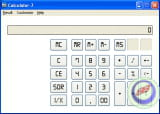Calculator-7

3 vote - 5.0 /5
The Calculator-7 is a software that acts like a pocket calculator and works under all versions of Windows and requires only 1MB of disk space. It can handle calculations up to 24 digits before the decimal point. It includes a function that calculates the numbers from a spreadsheet or a text editor. It can add, subtract, multiply, divide and calculate the percentage or square root. It also manages the calculations and figures as 1/X. It is also possible to modify the visual appearance such as color, size, policy or buttons. You can also customize the display of figures as well as the presentation of the decimal point of decimal numbers. It is intended for anyone using a calculator.